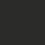# How to show two angles are equal

There are many ways to show two angles are equal. We usually try the first few techniques listed below, before the last couple.

• Using parallel lines;

• Using congruent triangles;

• Using isosceles triangles;

• Using parallelograms;

• Using similar triangles;

• Circle properties: Angles subtended by the same chord, external angles of cyclic quadrilaterals, alternate segment theorem;

• Via a third (or fourth) angle;

• Showing the two angles are the sum, difference, twice or half of other equal angles.

Exercises

$1$. In $\triangle{ABC}$, $\angle{A}=90^{\circ}$, $E$ is the foot of the perpendicular from $A$ to $BC$, $D$ is a point on $BC$ such that $BD=DC$ and $F$ is a point on $BC$ such that $\angle{BAF}=\angle{CAF}$. Show that $\angle{DAF}=\angle{FAE}$.

$2$. Let $ABCD$ be a parallelogram. Let $P$ be a point in the interior of $ABCD$ such that $\angle{BAP}=\angle{PCB}$. Show that $\angle{ABP}=\angle{ADP}$.

$3$. Let the angle bisectors of $\triangle{ABC}$, $AD$, $BE$, $CF$ intersect at $O$. Let $G$ be the foot of the perpendicular from $O$ to $BC$. Show that $\angle{BOD}=\angle{COG}$.

$4$. Let $ABCD$ be a quadrilateral such that $AD=BC$. Let $M$, $N$ be the respective midpoints of $AB$ and $DC$. Extend $AD$ and $MN$ to meet at $E$, $BC$ and $MN$ to meet at $F$. Show that $\angle{AEM}=\angle{BFM}$.Note by Victor Loh
6 years, 11 months ago

This discussion board is a place to discuss our Daily Challenges and the math and science related to those challenges. Explanations are more than just a solution — they should explain the steps and thinking strategies that you used to obtain the solution. Comments should further the discussion of math and science.

When posting on Brilliant:

• Use the emojis to react to an explanation, whether you're congratulating a job well done , or just really confused .
• Ask specific questions about the challenge or the steps in somebody's explanation. Well-posed questions can add a lot to the discussion, but posting "I don't understand!" doesn't help anyone.
• Try to contribute something new to the discussion, whether it is an extension, generalization or other idea related to the challenge.

MarkdownAppears as
*italics* or _italics_ italics
**bold** or __bold__ bold
- bulleted- list
• bulleted
• list
1. numbered2. list
1. numbered
2. list
Note: you must add a full line of space before and after lists for them to show up correctly
paragraph 1paragraph 2

paragraph 1

paragraph 2

[example link](https://brilliant.org)example link
> This is a quote
This is a quote
    # I indented these lines
# 4 spaces, and now they show
# up as a code block.

print "hello world"
# I indented these lines
# 4 spaces, and now they show
# up as a code block.

print "hello world"
MathAppears as
Remember to wrap math in $$ ... $$ or $ ... $ to ensure proper formatting.
2 \times 3 $2 \times 3$
2^{34} $2^{34}$
a_{i-1} $a_{i-1}$
\frac{2}{3} $\frac{2}{3}$
\sqrt{2} $\sqrt{2}$
\sum_{i=1}^3 $\sum_{i=1}^3$
\sin \theta $\sin \theta$
\boxed{123} $\boxed{123}$